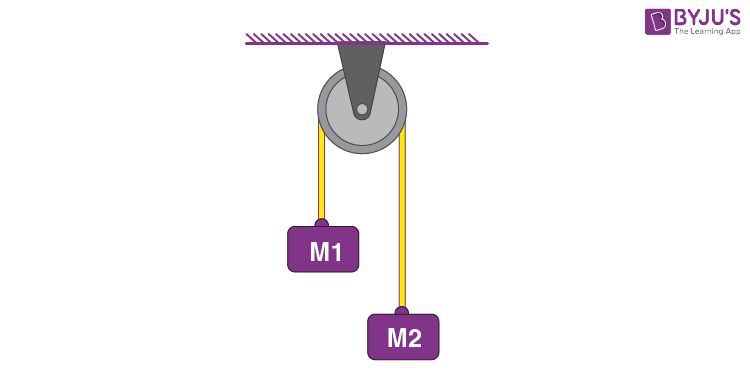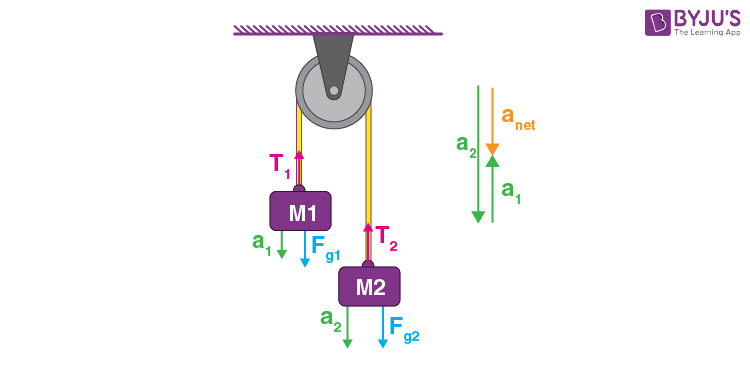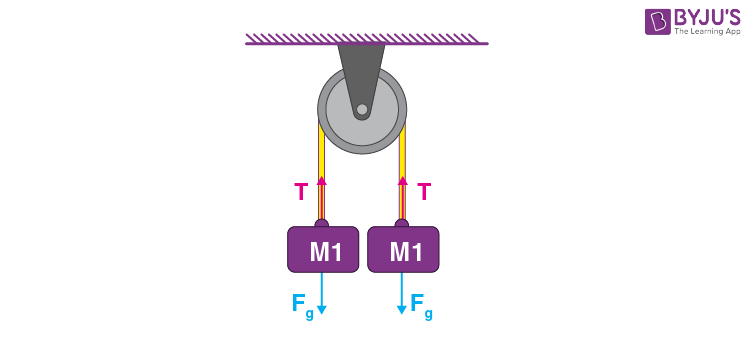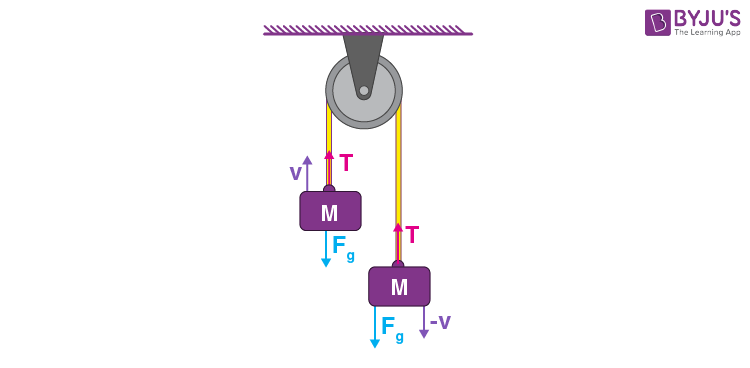Checkout JEE MAINS 2022 Question Paper Analysis : Checkout JEE MAINS 2022 Question Paper Analysis :

# Atwood Machine

The concept of the Atwood machine is based on the pulley system, which is widely used to study and analyse the mechanics in physics. In this article, you will read about the mechanism and working of the Atwood machine.

## What is Atwood’s Machine?

A simple device that runs over pulleys used to study the laws of motion & forces is known as Atwood’s machine. This machine is applicable in various scenarios in the study of mechanics. Basically, this machine is a perfect illustration of tension force, gravitational force and the downward force acting on a particular system of masses that are hung using a string and pulley arrangement.## Working of Atwood Machine

A simple apparatus that runs over a pulley is known as the Atwood machine. This device comprises two masses attached to a string and a pulley on which both the masses hang. For an ideal Atwood machine, it is best to assume that the pulley is frictionless and massless and the string to which the masses are attached is un stretchable and massless, having a constant length.

The diagram shown above is an ideal Atwood machine apparatus, and also it demonstrates one of the standard procedures to apply Newton’s second law of motion. We can draw free body diagrams for the masses in the Atwood machine, and for each free body diagram, we can represent the forces as a summation of equations.Using the standard labelling, let the smaller mass be m2, and the larger mass is m1, and since the forces are only acting in the vertical direction, we will use the summation equation for the forces along the y-axis only.

It can be clearly seen that only two forces are acting on each of the masses, that is, their own weight acting downwards and the tension in the string acting upwards.

Hence, the summation equation for forces on each mass can be written using the standard sign convention, the upward force is taken as positive, and the downward force is taken as negative.

T-m1g = m1a …. (1)

T-m2g = – m2a …. (2)

On solving (1) and (2) we get

a = g (m2 – m1)/ (m2 + m1)

Where, T = Tension in the string

a = magnitude of the acceleration of two masses

## Working of Atwood Machine in Different Cases

There are basically three different cases that can help you understand the working of an Atwood machine and the cases are as follows:

### 1. No Acceleration with Equal Masses

The diagram shows a situation of equilibrium. The force of gravity on each mass is equal because the two masses (M) are equal. The upward force will be termed tension (T) because it is opposing gravity in the string.Therefore, T will be equal to Fg for the system to stay in equilibrium. The net force can be written as 2Fg – 2T = 0; thus, there is no acceleration.

2T or 2Fg is the tension in the string. In this case, the tension can be expected to be the sum of the two downward forces because the string supports both masses.

### 2. Constant Velocity with Equal Masses

In this case, the total force in the system will be zero because constant velocity means no acceleration. So, this situation is different from the static situation.

There is no net velocity in this case because the velocity in the upward direction and the velocity in the downward direction have the same value or magnitude but in the opposite direction. Hence, there will be no acceleration too.This type of situation can be easily observed in our daily lives: For instance, if an equal and opposite constant force of friction is applied to an object that is a sliding object, then the object will move at constant velocity with zero acceleration.

Of course, the acceleration will exist if somehow the velocity of the system changes.

### Pulley System### 3. Acceleration with Unequal Masses

When the masses are unequal, then things get interesting. We will get acceleration in the downward direction of the heavier mass if we let the system go.

On each mass, the gravitational forces will now be unequal, and the vector sum of the two gravitational forces will be the net force in the direction of the heavier mass. That means that in the direction of M2, the net acceleration vector exists, as shown in the figure.For both masses, the total acceleration of the system is the same; the rate of acceleration of M1 upward is at the same rate as the acceleration of M2 downward since they are tied together.

The whole system cannot be treated as a single mass, M = M1 + M2.

 Related Articles

## Frequently Asked Questions – FAQs

### Define tension.

The force acting along the length of a particular medium such as the rope or a string is known as tension.

### What is an Atwood machine?

A simple device that runs over pulleys used to study the laws of motion & forces is known as Atwood’s machine.

### Define friction.

When two surfaces in contact move opposite to each other, then the resistance offered by them is known as friction.

### How many types of friction are there?

There are 4 types of friction:

• Static friction
• Sliding friction
• Rolling friction
• Fluid friction

### Explain in brief the working of the Atwood machine.

A simple apparatus that runs over a pulley is known as the Atwood machine. This device comprises two masses attached to a string and a pulley on which both the masses hang. For an ideal Atwood machine, it is best to assume that the pulley is frictionless and massless and the string to which the masses are attached is un stretchable and massless, having a constant length.

Stay tuned to BYJU’S for more such interesting and informative articles. Also, register to “BYJU’S – The Learning App” for loads of interactive, engaging Physics-related videos and unlimited academic assistance.